# Cartesian tree sorting

## 6 min

A fresh look at traditional concepts. Today there will be such a “Cartesian” which the school did not pass.The essence of the algorithm is that based on the array, the so-called Cartesian tree. And from the constructed Cartesian tree it is very easy to get all the elements in ascending or descending order.

At EDISON, we are always happy to join the beautiful.

One of our classic projects is Vivaldi Document Vault Diagnosticsthanks to which the simultaneous access to thousands of documents and works in the network library is optimized.

We love to sow the rational, the good, the eternal! 😉In the framework of this article, I decided to refrain from a detailed theory and will not explain what a Cartesian tree is, how to build it, and what operations are possible with it. This has already been done perfectly well before me – there is excellent material on Habré, where all these moments have been successfully and thoroughly covered – at the end of this lecture there is a section “Links” where you can go to these harastrastam. In the future, I will cover some points that are characteristic of this structure, but it is understood that you understand its specifics or, if interested, are ready to study it using the links below.

A Cartesian tree is a hybrid structure that combines the properties of a heap and a binary search tree. There are synonyms for this tree that will be used in the article:

deramide (deroar + feastmida),
trip (treaptree + heap)
and even ducha (dtree + kteaching)

Let’s take this array as an example:and take a look at what the binary search tree, heap and deramide look like for him.

### Binary search tree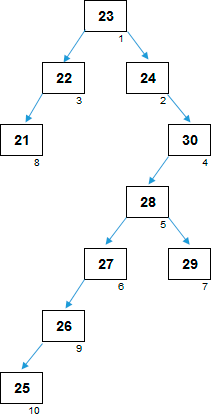The appearance of the tree greatly depends on how the elements of the array are initially located. Based on random data, a tree with a high degree of probability will turn out to be unbalanced, with a much larger number of levels compared to the optimal one. In our example, 7 levels formed. If the elements of the array were arranged, for example, in this order:

25, 28, 29, 23, 30, 27, 24, 22, 21, 26

the search tree would be balanced, with the minimum possible number of levels (= 4). Sorting using a balanced tree would have time complexity: O (n log n). But in the case of an unbalanced tree, it can degrade to O (n2).

In the previous habrastatia, sorting by a binary search tree was considered, as well as its interesting modification, which allows sorting with guaranteed time complexity O (n log n).

The more unbalanced the search tree is, the more costly it will be to traverse it.

On the other hand, storing data in the search tree is very convenient from an organizational point of view, it allows you to do a lot of operations with this data, including sorting.

A parent in a binary search tree is not necessarily more than a child. But at the same time, the left child is always smaller than the parent, the right child is always larger than the parent (or equal to it).

The maximum element of the array when building the search tree falls somewhere in the right subtree. To extract this maximum, the search tree must be completely circumvented.

### Binary heap

In an ordinary heap, parent-child relationships are very simply organized; they are tied to the element indices themselves.The figure is actually not a bunch yet, since such a tree, originally built on a random array, is not yet sorting. You need to go around each element and make a sift for it. As a result, the elements in the array are rebuilt so that each parent will be larger than its child: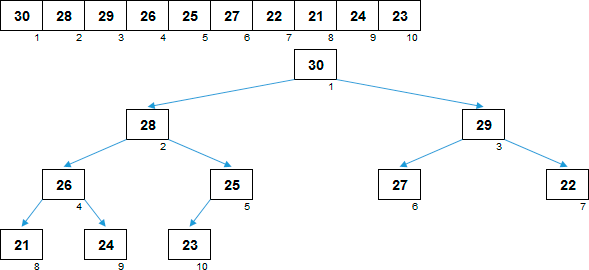The maximum element is at the root of the tree. Its location is known, you do not need to look for it. On the other hand, if you extract the maximum from the heap (and instead you have to put some other element of the array in the root of the tree), the heap ceases to be a sorting tree, it needs to be sifted again.

### Cartesian tree

And finally, the Cartesian tree. The relationships are established in such a way that, on the one hand, it is a bunch, if you look at values elements. All parents are more than their descendants: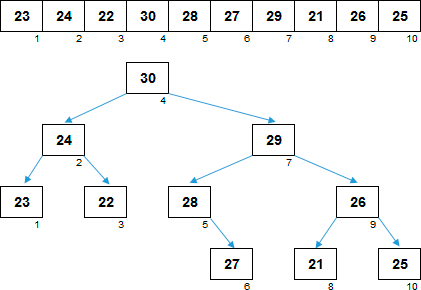On the other hand, the structure is a binary search tree, if you look at indices elements. For clarity, the values ​​of the elements in the nodes were removed from the image in the tree, only indexes were left. Each left descendant is smaller than the parent, each right descendant is larger than the parent: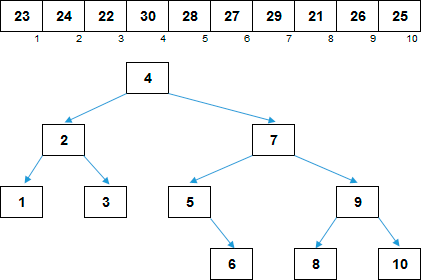On the one hand, we have quick access to the maximum element. Since this is a bunch, the maximum is at the root.

On the other hand, you can easily remove the maximum from the structure and work with the remaining data further. Since this is a binary search tree, removing the root is not expensive. Relatively small changes occur in the parent-child relationships, a new maximum hits the root of the Cartesian tree, and you can continue to work.

Each element is characterized by two numbers – the value of the element and the index of the element in the array. The index can be considered as the coordinate X, value – for Y, and the element itself should be interpreted as a point on the coordinate plane. If parents and descendants are connected by arrows, then we get something like this:The figure on the graph is completely isomorphic to the Cartesian tree just above.

## Cartesian tree sort :: Cartesian tree sort

The final algorithm:

• I. Based on an unsorted array, we construct a Cartesian tree:
• I.1. We sort through the elements of the array from left to right.
• I.1. If this is the first element of the array, then it is simply the very first node of the Cartesian tree.
• I.2. If this is not the first element of the array, consider this to be a Cartesian tree consisting of one element. We make an operation Merge this tree with the tree that is built from the previous elements.
• II. We delete the current maximum element from the tree, after which we restore the Cartesian tree.
• II.1. The current maximum element is the root of the tree.
• II.2. We remove the root from the tree, we transfer the maximum element to an additional array.
• II.3. After removing the root, the structure split into two Cartesian trees.
• II.4. For these two trees, we perform the operation Merge. The new current maximum is one of the roots of the joined trees.
• II.5. For a new high, repeat steps II.1-II.4 again.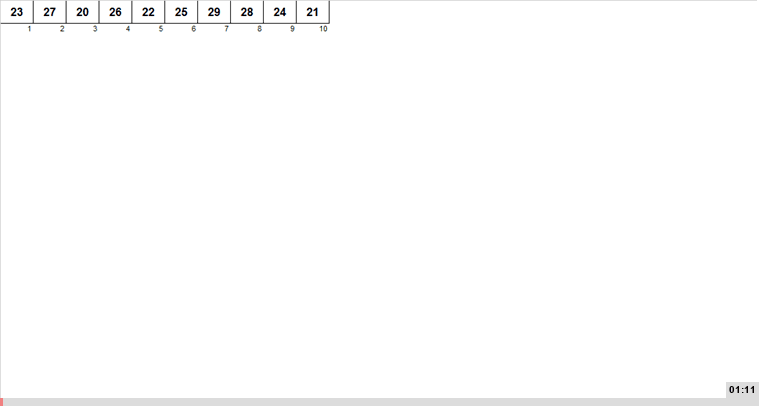Implementation in C ++:

``````/*
* c++ 11 code to construct a Cartesian tree. The method cartesianTreeSort
* will sort the contents of the array.
*/

#include
#include
#include

struct Node{
int value;
Node *left, *right;
Node *parent;

Node(){
value = 0;
parent = NULL;
left = NULL;
right = NULL;
}
};

// Used by priority queue
struct compare{
bool operator()(Node *left, Node *right){
return left->value > right->value;
}
};

class CartesianTree{
private:
// last pointer to keep track of last node added
Node *root, *last;

Node * findLowestNode(Node *node, int x){
if(node->value < x)
return node;
else if(node->parent != NULL)
return findLowestNode(node->parent, x);
else
return NULL;
}

public:
Node * getRoot(){
return root;
}

void addNode(int x){
Node *new_node = new Node;
new_node->value = x;
if(root == NULL){
last = new_node;
root = new_node;
return;
}
Node *z = findLowestNode(last, x);
if(z == NULL){
new_node->left = root;
root->parent = new_node;
root = new_node;
}
else{
new_node->left = z->right;
z->right = new_node;
new_node->parent = z;
}
last = new_node;
}

CartesianTree(std::vector ar){
root = NULL;
last = NULL;
// Call addNode function for each element of the array
for(auto x : ar){
addNode(x);
}
}

void InorderTraversal(Node *node){
// To print inorder traversal of the tree
if(node == NULL)
return;
InorderTraversal(node->left);
std::cout << node->value << ' ';
InorderTraversal(node->right);
}

// Function to sort and store values in array
void cartesianTreeSort(std::vector &sorted_ar){
// Initialize input array
sorted_ar.assign(0, 0);

// Initialize priority queue
std::priority_queue, compare> p_queue;
p_queue.push(root);
Node *temp = NULL;
while(!p_queue.empty()){
temp = p_queue.top();
p_queue.pop();
sorted_ar.push_back(temp->value);
if(temp->left){
p_queue.push(temp->left);
}
if(temp->right){
p_queue.push(temp->right);
}
}
}
};

int main(){
std::vector ar = {1, 14, 5, 0, 8};

CartesianTree tree(ar);
std::cout << "Inorder Traversaln";
tree.InorderTraversal(tree.getRoot());
std::cout << 'n';

std::vector sorted;
tree.cartesianTreeSort(sorted);
std::cout << "Sorted array isn";
for(auto x : sorted)
std::cout << x << ' ';
std::cout << 'n';
}``````

## Complexity

A Cartesian tree with minimal costs processes the sorted and almost sorted (and it does not matter, in ascending or descending) sections of the array. Here, for example, is the process for a reverse-ordered array: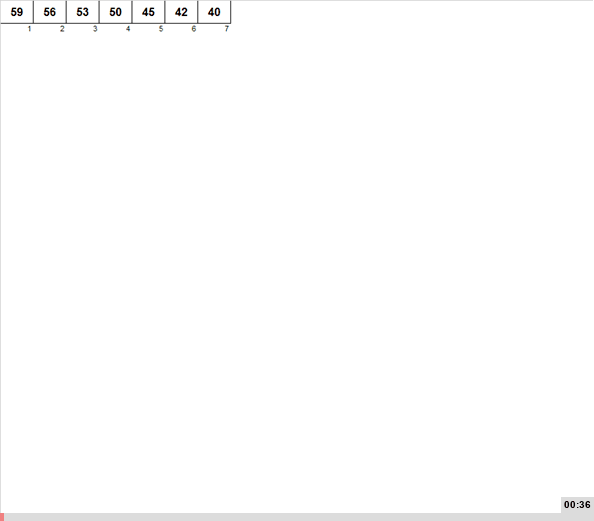The most expensive part of the algorithm is the Merge operation, which for each of n elements have to be done twice - first when building the deramide and then when disassembling it. On random data, a one-time Merge operation costs O (log n), but for elements located in the ordered regions of the array, the cost of the operation is O (1).

Thus, the average and worst time complexity of sorting is O (n log n)The best is O (n)

According to additional memory, things are worse - O (n) If the Cartesian tree was just a kind of heap, then the cost would be O (1). But the cartesian tree is also a binary search tree, which is why for everyone n elements have to separately store the relationship "parents-descendants."

In addition, the implementation in this form means that it is an external sorting - the sorted elements are collected in a separate array. And this is O (n) for additional memory.

## ReferencesCartesian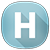Cartesian tree: Part 1, Part 2, Part 3

### Series Articles:

• Excel application AlgoLab.xlsm
• Exchange Sorts
• Insertion Sorts
• Sort by selection
• Heap Sorts: N-Pyramids
• Heap Sorts: Leonardo Numbers
• Heap sort: weak heap
• Bunch Sorts: Cartesian Tree
• Other heap sortings: mirror heap, mini-heap, sifting bottom-up
• Heap Sorts: Jung Heap
• Merge Sorts
• Sort by distribution
• Hybrid Sorting

Today’s Cartesian tree sorting has been added to the AlgoLab application, who uses it - update the excel file with macros. I recommend taking arrays with a small spread in the values ​​of the elements - otherwise the Cartesian plane will not fit on the screen.